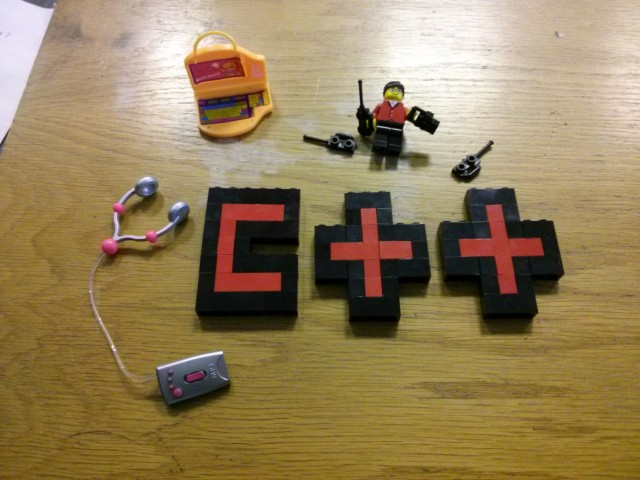# C++: Split a string in C++What’s the most elegant way to split a string in C++? The string can be assumed to be composed of words separated by whitespace.

(Note that I’m not interested in C string functions or that kind of character manipulation/access. Also, please give precedence to elegance over efficiency in your answer.)

The best solution I have right now is:

``````#include <iostream>
#include <sstream>
#include <string>
using namespace std;

int main()
{
istringstream iss(s);

do
{
string sub;
iss >> sub;
cout << "Substring: " << sub << endl;
} while (iss);

}``````

Ashwin

I use this to split string by a delim. The first puts the results in a pre-constructed vector, the second returns a new vector.

``````std::vector<std::string> &split(const std::string &s, char delim, std::vector<std::string> &elems) {
std::stringstream ss(s);
std::string item;
while (std::getline(ss, item, delim)) {
elems.push_back(item);
}
return elems;
}

std::vector<std::string> split(const std::string &s, char delim) {
std::vector<std::string> elems;
split(s, delim, elems);
return elems;
}``````

EDIT: Note that this solution does not skip empty tokens, so the following will find 4 items, one of which is empty:

``std::vector<std::string> x = split("one:two::three", ':');``

Evan Teran

Stack Overflow